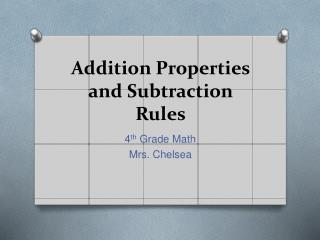DownloadDownload Presentation# Addition Properties and Subtraction Rules

Télécharger la présentation## Addition Properties and Subtraction Rules

- - - - - - - - - - - - - - - - - - - - - - - - - - - E N D - - - - - - - - - - - - - - - - - - - - - - - - - - -
##### Presentation Transcript

2. Vocabulary Say It! Addend Sum Commutative Property Associative property

3. Addend Any number being added together 8 + 7 = 15

4. Sum The answer to an addition problem 4 + 4 = 8

5. Commutative Property of Addition The order numbers are added does not change the sum. Ex. 4+1=5 1+4=5

6. Associative Property of Addition The way numbers are grouped when added does not change the sum. (5+2)+3 5+(2+3) 7 +3 5+ 5 10 10

7. Identity Property of Addition The sum of any number and 0 is the number. Ex. 8+0=8 0+8=8

8. Whiteboards: Check what you Know! Complete the number sentence and name the property. • 19 – X= 19 • (5+X)+2=5+(9+2) • 74+68=X+74 • 542+0= • (27+18)+13=27+ • (15+7)+23=15+ • 84+61=61+ Watch Brainpop: Commutative Property

9. Word Problem Words Addition • Altogether • In all • Sum • Add • Both • Total

10. Practice • In your Math Notebook: Math Book page 34 #s 8-13 and #s 25-28

11. ADDITION WITH REGROUPING J. Bennifield

12. NOW LET'S ADD THE HUNDREDS NOW LET'S ADD THE TENS BEGIN BY ADDING THE ONES Let's add this... 1 348 NO! We can only put the ones digit under the ones. YUP! It's 12! So, what do we do with the 1? What is 8 + 4? What is 4 + 4 + 1? RIGHT! We "carry" it to the tens! What is 3 + 4? YUP! It's 7! RIGHT! It's 9! + 444 Can we put the 12 under the ones? 9 2 7 12 J. Bennifield

13. Let's try another one... J. Bennifield

14. NOW LET'S ADD THE HUNDREDS NOW LET'S ADD THE TENS BEGIN BY ADDING THE ONES Let's add this... 1 RIGHT! We "carry" it to the tens! RIGHT! It's 5! So, what do we do with the 1? 215 NO! We can only put the ones digit under the ones. What is 2 + 2? YUP! It's 4! What is 1 + 1 + 3? YUP! It's 13! What is 5 + 8? + 238 Can we put the 13 under the ones? 5 3 4 13 J. Bennifield

15. Let's try one more... J. Bennifield

16. NOW LET'S ADD THE HUNDREDS NOW LET'S ADD THE TENS BEGIN BY ADDING THE ONES Let's add this... 1 1 495 Right! We "carry" it to the hundreds. NO! We can only put the ones digit under the ones. So, what do we do with the 1? No! We can only put the tens digit under the tens. RIGHT! We "carry" it to the tens! YUP! It's 10! What is 1 + 9 + 2? So what do we do with the 1? What is 5 + 5? RIGHT! It's 12! YUP! It's 6! What is 1 + 4 + 1? + 125 Can we put the 12 under the tens? Can we put the 10 under the ones? 6 12 2 0 10 J. Bennifield

17. Let's take 5 minutes to complete the 4 problems on your worksheet. J. Bennifield

18. 1 1 739 83 + 8 2 2 J. Bennifield

19. 1 462 81 + 5 4 3 J. Bennifield

20. 1 1 123 87 + 2 1 0 J. Bennifield

21. 1 28 643 + 6 7 1 J. Bennifield

23. To add decimals • Line up the decimal points and add the columns from right to left. • Place a decimal point in the answer directly below the other decimal points.

24. Example 1 0.64 + 0.39 Line up the decimal points. 0.64 + 0.39 __________________________ Add the columns. 0.64 + 0.39 __________________________ 1.03

25. Example 2 5.74 + 3.3 Line up the decimal points. 5.74 + 3.3 _____________________________ Add the columns. 5.74 + 3.3 _____________________________ 9.04

26. Subtraction Rules • When you subtract 0 from any number, the result is the number. Ex. 6-0=6 4-0=4 2. When you subtract any number from itself, the result is 0. Ex. 6-6=0 5-5=0

27. Subtraction Words Difference Fewer How many more How much more Left Less: Debra bought apples for \$3.20 and oranges for \$4.23. How much less did the apples cost? Minus Remains Subtract Words ending with "er"; higher, longer, faster, heavier, larger, shorter, slower, farther, etc. Example: Jean's apple weighs 100 grams, and Karen's apple weighs 80 grams. How much heavier is Jean's apple?

28. To subtract decimals • Line up the decimal points. • Subtract the columns from right to left, regrouping if necessary. • Place a decimal point in the answer directly below the other decimal points.

29. Example 1 0.86 – 0.49 Line up the decimal points 0.86 - 0.49 __________________________ Regroup the tenths. Subtract the columns. 0.86 - 0.49 __________________________ 16 7 0.37

30. Practice Time